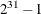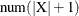### PROC OPTMODEL Statement

PROC OPTMODELoptions;

The PROC OPTMODEL statement invokes the OPTMODEL procedure. You can specify options to control how the optimization model is processed and how results are displayed. You can specify the following options (these options can also be specified in the RESET statement).

CDIGITS=number

specifies the expected number of decimal digits of accuracy for nonlinear constraints. The value can be fractional. PROC OPTMODEL uses this option to choose a step length when numeric derivative approximations are required to evaluate the Jacobian of nonlinear constraints. The default value depends on your operating environment. It is assumed that constraint values are accurate to the limits of machine precision.

See the section Automatic Differentiation for more information about numeric derivative approximations.

ERRORLIMIT=number | NONE

specifies the maximum number of error messages that can be displayed. Specifying a value of number in the range 1 tosets a specific limit. Specifying ERRORLIMIT=NONE removes any existing limit.

Note: Some errors abort processing immediately.

FD=FORWARD | CENTRAL

selects the method used to approximate numeric derivatives when analytic derivatives are unavailable. Most solvers require the derivatives of the objective and constraints. The methods available are as follows:

FD=FORWARD

use forward differences

FD=CENTRAL

use central differences

The default value is FORWARD. See the section Automatic Differentiation for more information about numeric derivative approximations.

FDIGITS=number

specifies the expected number of decimal digits of accuracy for the objective function. The value can be fractional. PROC OPTMODEL uses the value to choose a step length when numeric derivatives are required. The default value depends on your operating environment. It is assumed that objective function values are accurate to the limits of machine precision.

See the section Automatic Differentiation for more information about numeric derivative approximations.

INITVAR | NOINITVAR

selects whether or not to pass initial values for variables to the solver when the SOLVE statement is executed. INITVAR enables the current variable values to be passed. NOINITVAR causes the solver to be invoked without any specific initial values for variables. The INITVAR option is the default.

The LP and QP solvers always ignore initial values. The NLP solvers attempt to use specified initial values. The MILP solver uses initial values only if the PRIMALIN option is specified.

INTFUZZ=number

specifies the tolerance for rounding the bounds on integer and binary variables to integer values. Bounds that differ from an integer by at most number are rounded to that integer. Otherwise lower boundsare rounded up to the next greater integer and upper bounds are rounded down to the next lesser integer. The value of number can range between 0 and 0.5. The default value is 0.00001.

MAXLABLEN=number

specifies the maximum length for MPS row and column labels. The allowed range is 8 to 256, with 32 as the default. This option can also be used to control the length of row and column names displayed by solvers, such as those found in the LP solver iteration log. See also the description of the .label suffix in the section Suffixes.

MISSCHECK | NOMISSCHECK

enables detailed checking of missing values in expressions. MISSCHECK requests that a message be produced each time PROC OPTMODEL evaluates an arithmetic operation or built-in function that has missing value operands (except when the operation or function specifically supports missing values). The MISSCHECK option can increase processing time. NOMISSCHECK turns off this detailed reporting. NOMISSCHECK is the default.

MSGLIMIT=number | NONE

specifies the maximum number of non-error messages that can be displayed, including notes and warnings. Specifying a value of number in the range 0 tosets a specific limit. Specifying MSGLIMIT=NONE removes any existing limit.

PDIGITS=number

requests that the PRINT statement display number significant digitsfor numeric columns for which no format is specified. The value can range from 1 to 9. The default is 5.

PMATRIX=number

adjusts the density evaluation of a two-dimensional array to affect how it is displayed. The value number scales the total number of nonempty array elements and is used by the PRINT statement to evaluate whether a two-dimensional array is sparse or dense.Tables that contain a single two-dimensional array are printed in list form if they are sparse and in matrix form if they are dense. Any nonnegative value can be assigned to number; the default value is 1. Specifying a value for the PMATRIX= option that is less than 1 causes the list form to be used in more cases, while specifying a value greater than 1 causes the matrix form to be used in more cases. If the value is 0, then the list form is always used. See the section PRINT Statement for more information.

PRESOLVER=number | string

specifies a presolve string or its corresponding value number, as listed in Table 5.6.

Table 5.6: Values for the PRESOLVER= Option

number

string

Description

–1

AUTOMATIC

Applies presolver using default setting.

0

NONE

Disables presolver.

1

BASIC

Performs minimal processing, only substituting fixed variables and removing empty feasible constraints.

2

MODERATE

Applies a higher level of presolve processing.

3

AGGRESSIVE

Applies the highest level of presolve processing.

The OPTMODEL presolver tightens variable bounds and eliminates redundant constraints. In general, this improves the performance of any solver. The AUTOMATIC option is intermediate between the MODERATE and AGGRESSIVE levels.

Note: The OPTMODEL presolver is bypassed when using the LP, QP, or MILP solvers and when saving problem data with the SAVE MPS and SAVE QPS statements.

PRESTOL=number

provides a tolerance so that slightly infeasible constraints can be eliminated by the OPTMODEL presolver. If the magnitude of the infeasibility is no greater than, where X is the value of the original bound, then the empty constraint is removed from the presolved problem. OPTMODEL’s presolver does not print messages about infeasible constraints and variable bounds when the infeasibility is within the PRESTOL tolerance. The value of PRESTOL can range between 0 and 0.1; the default value is 1E12.

PRINTLEVEL=number

controls the level of listing output during a SOLVE command. The Output Delivery System (ODS) tables printed at each level are listed in Table 5.7. Some solvers can produce additional tables; see the individual solver chapters for more information.

Table 5.7: Values for the PRINTLEVEL= Option

number

Description

0

Disables all tables.

1

Prints Problem Summary and Solution Summary.

2

Prints Problem Summary, Solution Summary, Methods of Derivative Computation (for NLP solvers), Solver Options, Optimization Statistics, and solver-specific ODS tables.

For more details about the ODS tables produced by PROC OPTMODEL, see the section ODS Table and Variable Names.

PWIDTH=number

sets the width used by the PRINT statement to display numeric columns when no format is specified. The smallest value number can take is the value of the PDIGITS= option plus 7; the largest value number can take is 16. The default value is equal to the value of the PDIGITS= option plus 7.

VARFUZZ=number

specifies the smallest difference that is permitted by the OPTMODEL presolver between the upper and lower bounds of an unfixed variable. If the difference is smaller than number, then the variable isfixed to the average of the upper and lower bounds before it is presented to the solver. Any nonnegative value can be assigned to number; the default value is 0.